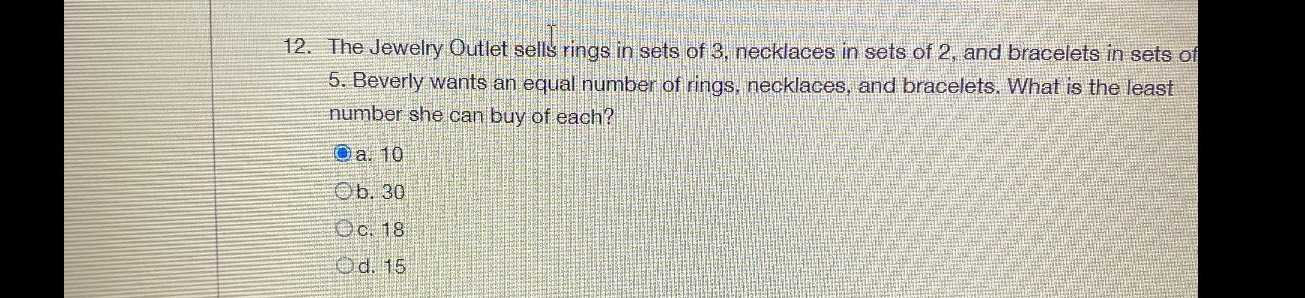### ¿Todavía tienes preguntas de matemáticas?

Pregunte a nuestros tutores expertos
Algebra
Pregunta12. The Jewelry Outlet sells rings in sets of $$3 ,$$ necklaces in sets of $$2 ,$$ and bracelets in sets of 5. Beverly wants an equal number of rings, necklaces, and bracelets. What is the least number she can buy of each?

a. $$10$$

b. $$30$$

c. $$18$$

d. $$15$$